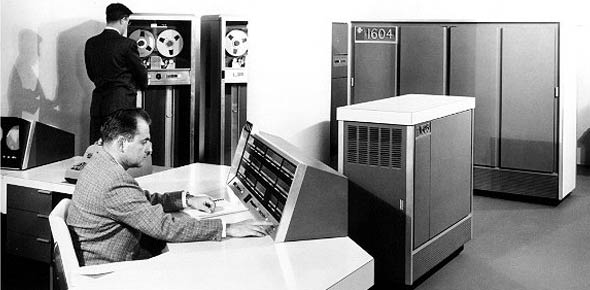# 2a553a 2011 Vol 3

100 Questions | Total Attempts: 127Settings2A553A 2011 VOL 3 URE CDC QUESTIONS

• 1.
(401) The polar axis is the
• A.

Axis about which the moon rotates

• B.

Axis about which the earth rotates

• C.

Circle equal distance from the poles

• D.

Circle formed by any plane that passes through the center of the earth

• 2.
What is formed on the surface of the Earth by any plane that passes through the center of the Earth and divides it into two equal parts?
• A.

Equator

• B.

Great circle

• C.

Parallel of latitude

• D.

Meridian of longitude

• 3.
Which system utilizes the equator as one fixed reference point and the Greenwich meridian as the other fixed reference point?
• A.

Aircraft landing

• B.

• C.

Instrument landing

• D.

Geographic coordinate

• 4.
(401) The prime meridian is the
• A.

Circle designated 0° latitude

• B.

Meridian designated the 180° meridian

• C.

Meridian passing through Greenwich, England

• D.

Circle equal distance between the north and south poles

• 5.
(401) From the prime meridian east to the international dateline, meridians are assigned values of
• A.

0° to 90° east

• B.

0° to 90° west

• C.

0° to 180° east

• D.

0° to 180° west

• 6.
(401) From the prime meridian west to the international dateline, meridians are assigned values of
• A.

0° to 90° east

• B.

0° to 90° west

• C.

0° to 180° east

• D.

0° to 180° west

• 7.
(401) In navigation, the primary reference is
• A.

• B.

90°

• C.

North

• D.

The equator

• 8.
(401) North on a compass rose is
• A.

• B.

90°

• C.

180°

• D.

270°

• 9.
(401) Relative bearing of an aircraft is the direction measured clockwise from
• A.

0° to the object

• B.

The object to true north

• C.

True north to the object

• D.

The aircraft heading to the object

• 10.
(402) The effect of wind on an aircraft is
• A.

Slight

• B.

Negligible

• C.

Significant

• D.

Unimportant

• 11.
(402) The sideward displacement of an aircraft by the wind is called
• A.

Yaw

• B.

Drift

• C.

Deviation

• D.

Groundspeed

• 12.
(402) The rate of movement of an aircraft relative to the Earth’s surface is called
• A.

Drift

• B.

Velocity

• C.

Airspeed

• D.

Groundspeed

• 13.
(402) What is used to correct true heading and allow the aircraft to make good the desired true course?
• A.

Drift correction

• B.

Wind disbursement

• C.

Airspeed correction

• D.

Ground speed correction

• 14.
(402) A vector diagram that shows the effect of the wind on the flight of an aircraft is called the
• A.

Flight path

• B.

Wind triangle

• C.

• D.

Destination chart

• 15.
(402) On navigation charts, the lines that connect points of equal magnetic variation are called
• A.

Agonic

• B.

Isogonic

• C.

Variation lines

• D.

Deviation lines

• 16.
(403) If the airspeed of the aircraft is too fast, a falling bomb
• A.

Hits the target

• B.

Overshoots the target

• C.

Falls short of the target

• D.

Falls to the side of the target

• 17.
(403) Air resistance causes a falling bomb to
• A.

Lag

• B.

• C.

Fall rapid

• D.

Fall unpredictably

• 18.
(403) A head wind causes a falling bomb to
• A.

Hit the target

• B.

Overshoot the target

• C.

Fall short of the target

• D.

Fall left or right of the target

• 19.
(403) A cross wind causes a falling bomb to
• A.

Hit the target

• B.

Overshoot the target

• C.

Fall short of the target

• D.

Fall left or right of the target

• 20.
(403) The configuration of the onboard weapons can be described in the
• A.

Action point table

• B.

Release table

• C.

Gravity table

• D.

Stores table

• 21.
(403) The preplanned flight route can be described in the
• A.

Action point table

• B.

Release table

• C.

Gravity table

• D.

Stores table

• 22.
(404) Which is not a basic inertial navigation system component?
• A.

Accelerometer

• B.

Computer

• C.

Integrator

• D.

Indicator

• 23.
(404) The changing of position with respect to some other body is called
• A.

Speed

• B.

Motion

• C.

Velocity

• D.

Reference

• 24.
(404) Which is used to maintain accelerometer orientation in the inertial navigation system?
• A.

Summing unit

• B.

Computer

• C.

Integrator

• D.

Platform

• 25.
(405) What line replaceable unit consists of a gyro-stabilized platform, platform electronics,navigation computer, input/output functions, and power supply?
• A.

• B.

Doppler velocity sensor

• C.

Terrain-following avionics control unit

• D.Back to top